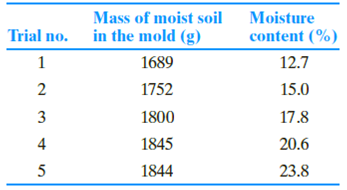Chapter 6, Problem 6.9PPrinciples of Geotechnical Enginee...

9th Edition
Braja M. Das + 1 other
ISBN: 9781305970939

Solutions

Chapter
SectionPrinciples of Geotechnical Enginee...

9th Edition
Braja M. Das + 1 other
ISBN: 9781305970939
Textbook Problem

A standard Proctor test was conducted on a silty clay soil collected from a proposed construction site. The results are shown in the following table.a. Determine the maximum dry density (kg/m3) of compaction and the optimum moisture content. Given: Mold volume = 943.3 cm3.b. If specification calls for 99% relative compaction in the field, what would be the field dry density and the range of acceptable moisture content?Refer to the silty clay soil at the construction site in Problem 6.8. As part of a quality control program, the field inspection engineer conducted a sand cone test to determine the field density. The following data were recorded using the sand cone method.• Calibrated dry density of Ottawa sand = 1667 kg/m3• Mass of Ottawa sand to fill the cone = 0.117 kg• Mass of jar + cone + sand (before use) = 6.1 kg• Mass of jar + cone + sand (after use) = 2.83 kg• Mass of moist soil from hole = 3.35 kg• Moisture content of moist soil = 16.1%a. Determine the dry unit weight of compaction in the field.b. What is the relative compaction in the field?c. Was the compaction specification stated in Problem 6.8 met?

(a)

To determine

The dry unit weight of compaction in the field.

Explanation

Given information:

The calibrated dry density of Ottawa sand ρd(sand) is 1,667kg/m3.

The mass of Ottawa sand (Msand) is 0.117 kg.

Mass of jar, cone, and sand before use (M)b is 6.1 kg.

Mass of jar, cone, and sand after use (M)a is 2.83 kg.

Mass of moist soil from hole Msoil is 3.35 kg.

The moisture content of the moist soil w 16.1 %.

Calculation:

Determine the mass of sand used to fill the hole and cone using the relation.

Mh+c=(M)b(M)a

Substitute 6.1 kg for (M)b and 2.83 kg for (M)a.

Mh+c=6.12.83=3.27kg

Determine the mass of sand used to fill the hole using the relation.

Mh=Mh+cMsand

Substitute 3.27 kg for Mh+c and 0.117 kg for Msand.

Mh=3.270.117=3.153kg

Determine volume of the hole using the relation.

Vh=Mhρd(sand)

Substitute 3.153 kg for Mh and 1,667kg/m3 for ρd(sand).

Vh=3

(b)

To determine

The relative compaction in the field.

(c)

To determine

Compare the relative compaction in the field with the required relative compaction.

Still sussing out bartleby?

Check out a sample textbook solution.

See a sample solution

The Solution to Your Study Problems

Bartleby provides explanations to thousands of textbook problems written by our experts, many with advanced degrees!

Get Started

What are the basic characteristics of a NoSQL database?

Database Systems: Design, Implementation, & Management

List two applications of Acme threads.

Precision Machining Technology (MindTap Course List)

Is batch processing still relevant? Why or why not?

Systems Analysis and Design (Shelly Cashman Series) (MindTap Course List)

Which form of SHA was developed by private designers?

Network+ Guide to Networks (MindTap Course List)